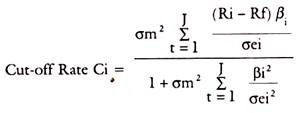# Portfolio Theories: Sharp Optimization Model

Markowitz Model had serious practical limitations due to the rigours involved in compiling the expected returns, standard deviation, variance, covariance of each security to every other security in the portfolio. Sharpe Model has simplified this process by relating the return in a security to a single Market index. Firstly, this will theoretically reflect all well traded securities in the market. Secondly, it will reduce and simplify the work involved in compiling elaborate matrices of variances as between individual securities.

If thus the market index is used as a surrogate for other individual securities in the portfolio, the relation of any individual security with the Market index can be represented in a Regression line or characteristic line. This is drawn below, with the excess return on the security on the y-axis and excess return on the Market Portfolio on the x-axis.

The equation of the characteristic line is

Ri – Rf = a + βim (Rm – Rf) + ei

Ri is the holding period return on security i

Rf is the riskless rate of interest

Alpha is the vertical intercept on y-axis representing the return on the security when only unsystematic risk is considered and systematic risk is measured by Beta. ci is the residual component, not captured by the above variables.The sharpe equation is as follows:

Rj = αj + βj + ej

Where αj is some constant, say risk free return

βj is the Beta which is a risk measure of the market called systematic risk

I is the value or return on the stock index.

ej is the residual factor which cannot be specified.

### Optimal Portfolio of Sharpe Model:

This optimal portfolio of Sharpe is called the Single Index Model. The optimal portfolio is directly related to the Beta. If Ri is expected return on stock i and Rf is Risk free Rate, then the excess return = Ri – Rf This has to be adjusted to Bi, namely,

Ri – Rf/βi which is the equation for ranking Stocks in the order of their return adjusted for risk.

The method involves selecting a cut-off rate for inclusion of securities in a portfolio. For this purpose, excess return to Beta ratio given above has to be calculated for each stock and rank them from highest to lowest. Then only those securities which have Ri – Rf/βi, greater than cut-off point, fixed in advance can be selected.

The basis for finding the cut-off Rate Ci is as follows:

Basis for Cut-off Rate:

For a portfolio of i stocks, Ci is given by cut-off rate:σm2 = variance in the market Index

σei2 = variance in the Stock movement in unsystematic Risk.

Ri, Rf, Bi have the same meanings as referred to above.

## One thought on “Portfolio Theories: Sharp Optimization Model”

error: Content is protected !!